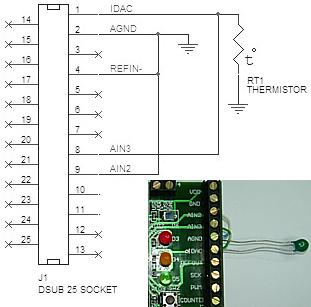The following information is for the EMANT380

# Measure Temperature using the Thermistor

Learn:

• Analog Output
• Thermistor

A thermistor can be used to measure temperature. Thermistors are widely used in industrial applications because of their sensitivity, small size, ruggedness and low cost. Thermistors have an electrical resistance that varies non-linearly with temperature. The R-T characteristics of most thermistors can be described by the Steinhart-Hart equation:

1/T = A + B*(Ln R) + C*(Ln R)3

T is the absolute temperature (in Kelvin) and A, B, and C are constants which can be determined by measuring three sets of resistance and temperature values during calibration.

Most thermistors have a negative temperature coefficient (NTC), their resistance decreases with increasing temperature. Thermistors are specified according to its nominal resistance at 25 oC and commonly available thermistors range from 250 ohms to 100 kohms

The thermistor that we are using has the following characteristics

• Nominal resistance @ 25 oC: 10 kohms
• negative temperature coefficient (NTC)
• Steinhart-Hart equation parameters:
• A= 0.001129148
• B= 0.000234125
• C= 8.76741E-8

As the DAQ module Analog Input measures only voltage, we will need to provide a current source to convert the resistance to voltage. The EMANT380 has an 8 bit current DAC (digital to analog converter). As the DAC has 8 bits resolution, we can drive the resistance from 0 to 1mA in 255 steps with increments of about 39uA. In our exercise, we will drive 0.1mA into the thermistor. As the thermistor has a nominal value of 10 kohm at 25 oC, at this temperature the voltage across the thermistor will be (0.1mA * 10 kohm) = 1V.• Connect the thermistor to the Light Application Adaptor screw terminals labeled IDAC and AGND
• Connect a wire from IDAC to AIN3
• Connect a wire from AGND to AIN2

import emant import math A = 0.001129148 B = 0.000234125 C = 0.0000000876741 m = emant.Emant300() m.Open("00:06:66:00:a1:D8") print m.HwId() m.WriteAnalog(0.1) volt, binval = m.ReadAnalog(emant.Emant300.AIN3,emant.Emant300.AIN2) R = volt / 0.0001 temp = 1 / ( A + B * math.log(R) + C * pow(math.log(R), 3) ) temp = temp - 273 print R print temp m.Close()

## Code Explained

m.WriteAnalog(0.1)

The parameter 0.1 (variable is double data type) sets the current output to 0.1 mA. Value must be between 0 to 1 mA

temp = 1 / ( A + B * math.log(R) + C * pow(math.log(R), 3) )

math.log is one of the methods from the math Class. The math Class provides constants and methods for trigonometric, logarithmic, and other common mathematical functions.

temp = temp - 273

The temperature is converted from oK to oC